LinUCB 浅析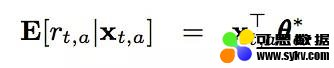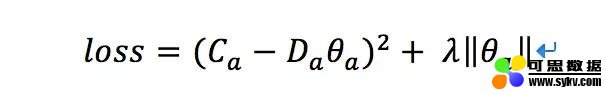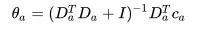可思数据-人工智能资讯平台sykv.com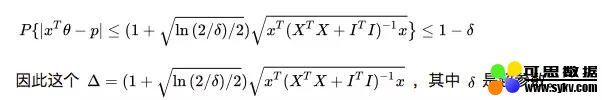可思数据-AI,sykv.com人工智能,深度学习,机器学习,神经网络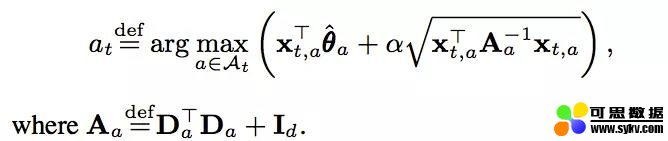可思数据-www.sykv.cn,sykv.com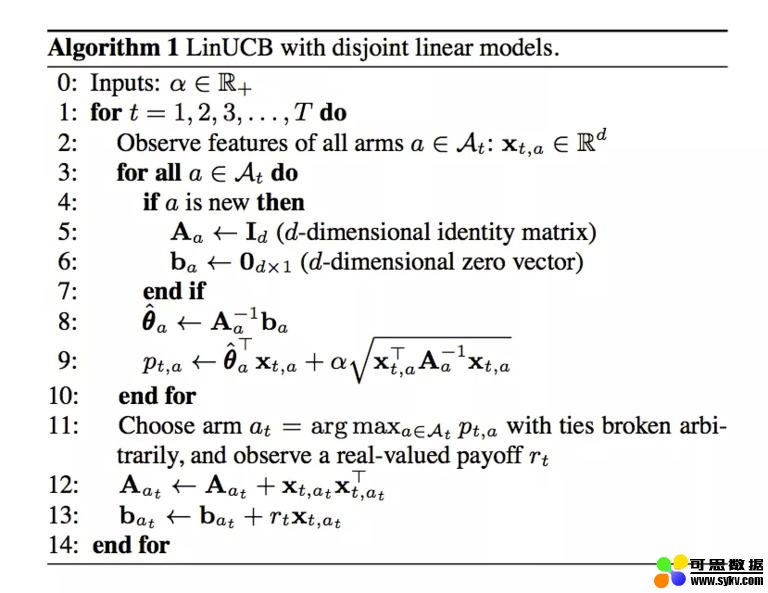• 本文地址：http://www.6aiq.com/article/1547825582955
• 本文版权归作者和AIQ共有，欢迎转载，但未经作者同意必须保留此段声明，且在文章页面明显位置给出
• 知乎专栏 点击关注

2、linUCB 代码实战

设定超参数和矩阵

self.alpha = 0.25
self.r1 = 0.6
self.r0 = -16
self.d = 6  # dimension of user features
可思数据-AI,sykv.com智能驾驶,人脸识别,区块链,大数据

self.Aa = {} # Aa : collection of matrix to compute disjoint part for each article a, d*d
self.AaI = {} # AaI : store the inverse of all Aa matrix

self.ba = {} # ba : collection of vectors to compute disjoin part, d*1
self.theta = {}

可思数据sykv.com

初始化矩阵

def set_articles(self,art):
for key in art:
self.Aa[key] = np.identity(self.d) # 创建单位矩阵
self.ba[key] = np.zeros((self.d,1))

self.AaI[key] = np.identity(self.d)
self.theta[key] = np.zeros((self.d,1))

计算推荐结果

def recommend(self,timestamp,user_features,articles):
xaT = np.array([user_features]) # d * 1
xa = np.transpose(xaT)

AaI_tmp = np.array([self.AaI[article] for article in articles])
theta_tmp = np.array([self.theta[article] for article in articles])
art_max = articles[np.argmax(np.dot(xaT,theta_tmp) + self.alpha * np.sqrt(np.dot(np.dot(xaT,AaI_tmp),xa)))]

self.x = xa
self.xT = xaT

self.a_max = art_max
return self.a_max

更新矩阵信息

def update(self,reward):
if reward == -1:
pass
elif reward == 1 or reward == 0:
if reward == 1:
r = self.r1
else:
r = self.r0

self.Aa[self.a_max] += np.dot(self.x,self.xT)
self.ba[self.a_max] += r * self.x
self.AaI[self.a_max] = np.linalg.inv(self.Aa[self.a_max])
self.theta[self.a_max] = np.dot(self.AaI[self.a_max],self.ba[self.a_max])

else:
# error

结语

1）由于加入了特征，所以收敛比 UCB 更快（论文有证明）； 可思数据-www.sykv.cn,sykv.com

2）特征构建是效果的关键，也是工程上最麻烦和值的发挥的地方； 本文来自可思数据(sykv.com)，转载请联系本站及注明出处

3）由于参与计算的是特征，所以可以处理动态的推荐候选池，编辑可以增删文章； 内容来自可思数据sykv.com

4）特征降维很有必要，关系到计算效率。

5）是一种在线学习算法。

参考文献

1、https://arxiv.org/pdf/1003.0146.pdf 可思数据sykv.com,sykv.cn

2、https://zhuanlan.zhihu.com/p/35753281 可思数据-www.sykv.cn,sykv.com

3、https://blog.csdn.net/legendavid/article/details/71082124 可思数据sykv.com,sykv.cn

4、https://zhuanlan.zhihu.com/p/32382432